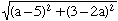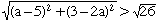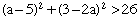Dear Sir/Madam, Could you answer this problem as soon as possible please? For what values of a is the distance between P(a, 3) and Q(5, 2a) greater than the square root of 26. Hi, Use the distance function find the distance between P and Q, that isHence you want to find all values of a such thatSince the quantities under each root are positive this is equivalent to solvingAdd -26 to both sides, expand the left side and simplify. You then have an inequality to solve with a quadratic on the left and zero on the right. You may find it easier to first replace the inequality by an equality and solve. Cheers, Penny Go to Math Central